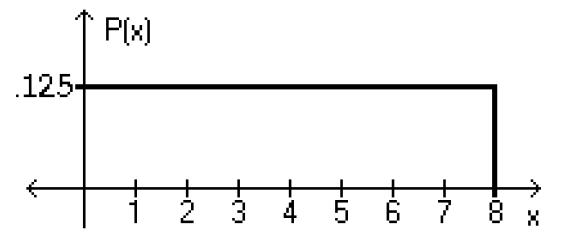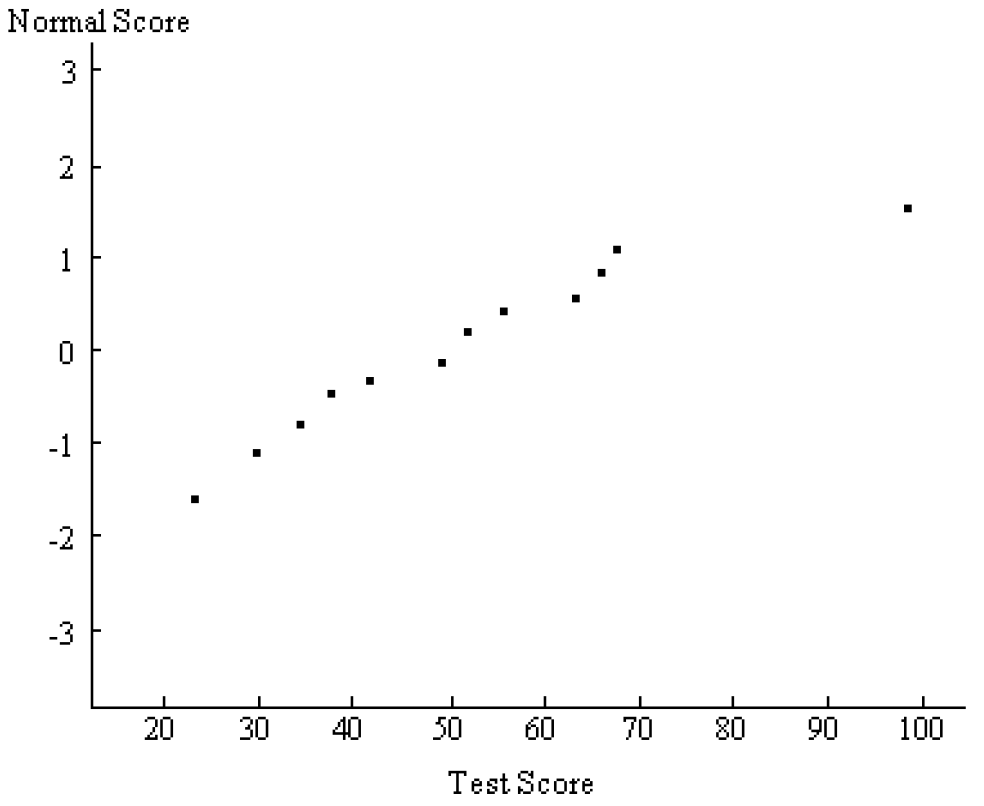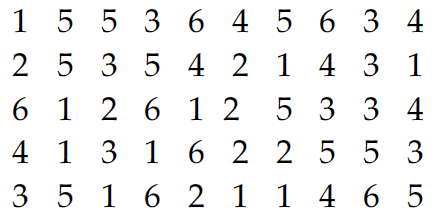Jan 13, 2017

# Normal Probability Distribution — practice test 6C

20 cards
Problems with solutions in Elementary Statistics — The Standard Normal Distribution, Applications of Normal Distributions, Sampling Distributions and Estimators, The Central Limit Theorem, Normal as Approximation to Binomial, Assessing Normality

Directions:

• Provide an appropriate response.
• 1. According to data from the American Medical Association, 10% of us are left-handed. Suppose groups of 500 people are randomly selected. Find the provability that at least 80 are left-handed. Describe the characteristics of this problem which help you to recognize that the problem is about a binomial distribution which you are to solve by estimating with the normal distribution. (Assume that you would not use a computer, a table, or the binomial probability formula.)

The problem statement has a stated percent, 10%, which corresponds to the p value, and a fixed number of trials, 500, which corresponds to n. No mean or standard deviation is given, and they are to be computed using the formula μ=n*p and σ=sq. root of n*p*q. Also, the normal approximation to the binomial distribution is appropriate, since both np and nq are greater than 5. P=0.0001.

• Using the following uniform density curve, answer the question.
• 2.What is the probability that the random variable has a value greater than 1.6?
1. 0.7500
2. 0.8000
3. 0.9250
4. 0.6750

• Assume that the weight loss for the first month of a diet program varies between 6 pounds and 12 pounds, and is spread evenly over the range of possibilities, so that there is a uniform distribution. Find the probability of the given range of pounds lost.
• 3. Between 9.5 pounds and 11 pounds
1. ¼
2. 1/3
3. ½
4. ¾

• If Z is a standard normal variable, find the probability.
• 4. The probability that Z lies between 0.7 and 1.98
1. 1.7341
2. –0.2181
3. 0.2181
4. 0.2175

• 5. P(Z < 0.97)
1. 0.8340
2. 0.8315
3. 0.1160
4. 0.8078

• The Precision Scientific Instrument Company manufactures thermometers that are supposed to give readings of 0°C at the freezing point of water. Tests on a large sample of these thermometers reveal that at the freezing point of water, some give readings below 0°C (denoted by negative numbers) and some give readings above 0°C (denoted by positive numbers). Assume that the mean reading is 0°C and the standard deviation of the readings is 1.00°C. Also assume that the frequency distribution of errors closely resembles the normal distribution. A thermometer is randomly selected and tested. Find the temperature reading corresponding to the given information.
• 6. If 9% of the thermometers are rejected because they have readings that are too low, but all other thermometers are acceptable, find the temperature that separates the rejected thermometers from the others.
1. –1.39°
2. –1.45°
3. –1.26°
4. –1.34°

• 7. A quality control analyst wants to examine thermometers that give readings in the bottom 7%. Find the reading that separates the bottom 7% from the others.
1. –1.89°
2. –1.48°
3. –1.63°
4. –1.75°

• Assume that X has a normal distribution, and find the indicated probability.
• 8. The mean is μ = 15.2 and the standard deviation is σ = 0.9.
Find the probability that X is greater than 16.1.
1. 0.8413
2. 0.1357
3. 0.1550
4. 0.1587

• 9. The mean is μ = 15.2 and the standard deviation is σ = 0.9.
Find the probability that X is between 14.3 and 16.1.
1. 0.1587
2. 0.3413
3. 0.6826
4. 0.8413

• Solve the problem.
• 10. The serum cholesterol levels for men is one age group are normally distributed with a mean of 178.1 and a standard deviation of 40.8. All units are in mg/100ml. Find the two levels that separate the top 9% and the bottom 9%.
1. 165.0 mg/100mL and 191.16 mg/100mL
2. 123.4 mg/100mL and 232.8 mg/100mL
3. 161.4 mg/100mL and 194.8 mg/mL
4. 107.1 mg/100mL and 249.1 mg/100mL

• Find the indicated probability.
• 11. The incomes of trainees at a local mill are normally distributed with a mean of \$1100 and a standard deviation \$150. What percentage of trainees earn less than \$900 a month?
1. 35.31%
2. 40.82%
3. 9.18%
4. 90.82%

• Provide an appropriate response.
• 12. A poll of 1400 randomly selected students in grades 6 through 8 was conducted and found that 30% enjoy playing sports. Is the 30% result a statistic or a parameter? Explain.

Statistic, because it is calculated from a sample, not a population.

• List the different possible samples, and find the mean of each of them.
• 13. Flood insurance policies sold in the last three days by a new agent were 2, 6, and 7. Assume then samples of size 2 are randomly selected with replacement from this population of three values.

Possible samples: 2–2; 2–6; 2–7; 6–2; 6–6; 6–7; 7–2; 7–6; 7–7.
Means: 2, 4, 4.5, 4, 6, 6.5, 4.5, 6.5, 7

• Solve the problem.
• 14. For women aged 18-24, systolic blood pressures (in mm Hg) are normally distributed with a mean of 114.8 and a standard deviation of 13.1. If 23 women aged 18-24 are randomly selected, find the probability that their mean systolic blood pressure is between 119 and 122.
1. 0.9341
2. 0.0833
3. 0.3343
4. 0.0577

• 15. A study of the amount of time it takes a mechanic to rebuild the transmission for a 1992 Chevrolet Cavalier show that mean is 8.4 hours and the standard deviation is 1.8 hours. If 40 mechanics are randomly selected, find the probability that their mean rebuild time exceeds 7.7 hours.
1. 0.9634
2. 0.9931
3. 0.8531
4. 0.9712

• Use the continuity correction and describe the region of the normal curve that corresponds to the indicated binomial probability.
• 16. The probability of at least 53 boys
1. The area to the left of 52.5
2. The area to the right of 53.5
3. The area to the right of 52.5
4. The area to the right of 53

• For the binomial distribution with the given values for n and p, state whether or not it is suitable to use the normal distribution as an approximation.
• 17. n = 18, p = .2
1. Normal approximation is suitable.
2. Normal approximation is not suitable.

• Estimate the indicated probability by using the normal distribution as an approximation to the binomial distribution.
• 18. The probability that a radish seed will germinate is 0.7. Estimate the probability that of 140 randomly selected seeds, exactly 100 will germinate.
1. 0.0769
2. 0.0669
3. 0.9331
4. 0.0679

• Solve the problem.
• 19. A normal probability plot is given below for a sample of scores on an aptitude test. Use the plot to assess the normality of scores on this test. Explain your reasoning.